Latest Banking jobs   »

# Reasoning Quizzes Quiz For IRDA AM 2023- 19th April

Directions (1-3): Study the following information carefully and answer the questions given below:
S is 5m to the west of K. I is 4m to the north of K. P is 5m to the east of I. J is 4m to the south of P. F is 2m to the east of J.

Q1. What is the shortest distance between point I and point S?
(a) 32m
(b) 41m
(c) √31m
(d) √41m
(e) None of these

Q2. What is the direction of point F with respect to point I?
(a) South east
(b) North
(c) South west
(d) West
(e) None of these

Q3. What is the shortest distance between point P and point F ?
(a)√21m
(b)2m
(c)2√5m
(d)3m
(e)None of these

Direction (4-6): Study the following information carefully and answer the questions given below:
Point J is towards the north of point K. point K is towards the west of point L. Point L is towards the north of point M. Point M is towards the east of point O. Point O is towards the north of point N. Where, JK=2KL=2LM=2MO=ON=10m.

Q4. Which of the following point lies on a straight line?
(a)J,K,M
(b)K,O,L
(c)N,M,L
(d)N,O,M
(e)J,K,O

Q5. What is the total distance between J and N?
(a)35m
(b)25m
(c)45m
(d)55m
(e)65m

Q6. Point M is in which direction with respect to K?
(a)North-east
(b)South-east
(c)South-west
(d)North-west
(e)North

Direction (7-9): Study the following information carefully and answer the questions given below:
Hemant starts walking in the north direction from his house which is at point O. After walking 15m he turns towards his left and walks another 15m. He then turns towards his right and walks 20m to reach his office at point K. Heena starts walking from point O in the south direction. After walking 20m she turns towards her left and walks 15m. After that she turns towards her left and walks another 15m to reach her office at point J.

Q7. What is the shortest distance between point K and point J?
(a)50m
(b)60m
(c)53m
(d)55m
(e)65m

Q8. In which direction is point J with respect to point O?
(a)South-west
(b)North-east
(c)South
(d)South-east
(e)North-west

Q9. If point M is towards east of point K and towards north of point J, then what is the shortest distance between M and J?
(a)40m
(b)45m
(c)35m
(d)Cannot be determined
(e)50m

Direction (10-12): Study the following information carefully and answer the questions given below:
Point A is 25m west of point B. Point B is 30m north of point C. Point D is 15m west of point C. Point E is 30m south of point D. Point G is 20m east of point E.

Q10. What is the shortest distance between point C and point E?
(a)√1225m
(b)25√5m
(c)10√5m
(d)15√5m
(e)1125m

Q11. Point G is in which direction with respect to point B?
(a)South
(b)South-west
(c)South-east
(d)North
(e)North-east

Q12. If point K is south of point A and west of point E, then what is the shortest distance between G and K?
(a)20m
(b)25m
(c)40m
(d)50m
(e)None of these

Direction (13-15): Study the following information carefully and answer the questions given below.
D is 5m to the west of E. F is 2m to the west of C. B is 4m to the south of C. A is 5m to the west of B. E is 4m to the south of A.

Q13. What is the shortest distance between point E and point B?
(a)√41m
(b)√43m
(c)5m
(d)4√3m
(e) None of these

Q14. What is the direction of point C with respect to point D ?
(a)North
(b)North east
(c)West
(d)South
(e)South east

Q15. What is the total distance between the point E and Point F?
(a)11m
(b)14m
(c)15m
(d)12m
(e)16m

Solutions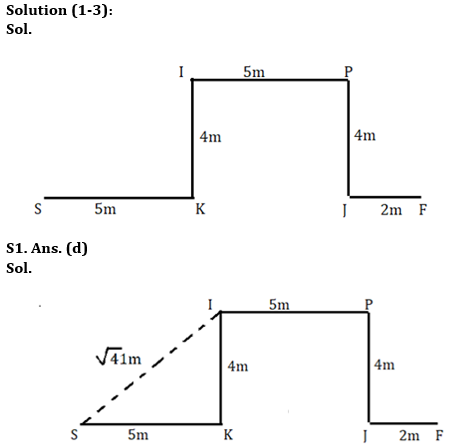S2. Ans. (a)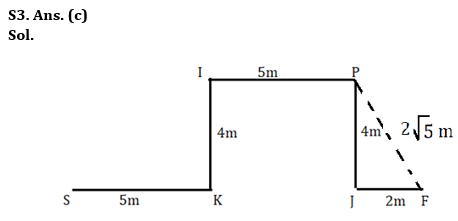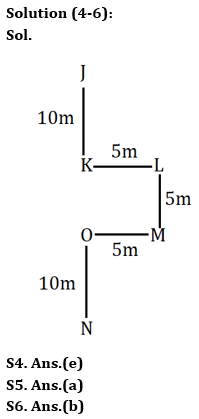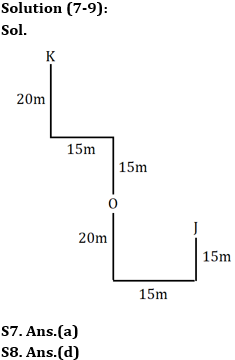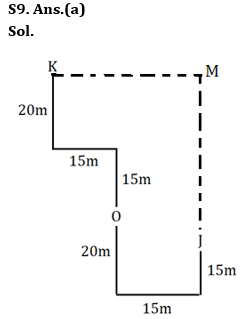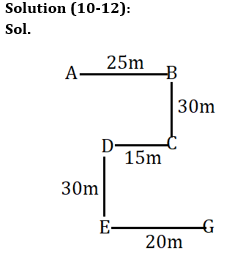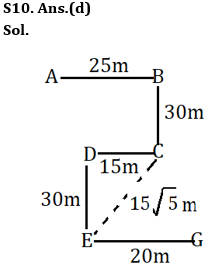S11. Ans.(c)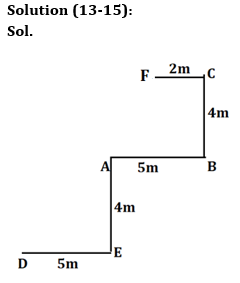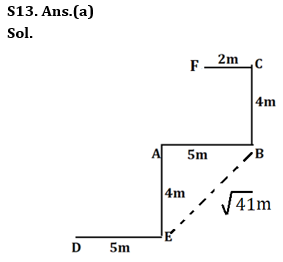S14. Ans.(b)
S15. Ans.(c)## FAQs

### How many sections are there in the IRDAI exam?

There are 4 sections in the IRDAI assistant manager online preliminary exam. They are Reasoning, English Language, General awareness, Quantitative aptitude.

#### Congratulations!Union Budget 2023-24: Free PDF